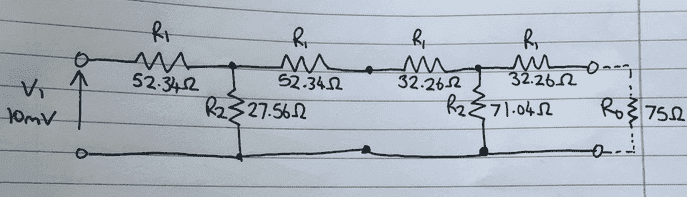# V2 of two symmetrical T attenuator pads in cascade

## Homework Statement

http://imgur.com/qBXvrGzAbove is a sketch of the circuit, a two stage symmetrical attenuator consisting of two symmetrical T attenuator pads connected in cascade, with the values that I have worked out for the resistors.

The reduction is 15dB in the first stage, and 8dB in the second stage, giving a total reduction of 23dB.

Given an input Voltage of 10mV, I must work out the output voltage. R_0 = 75 Ohms

## The Attempt at a Solution

I am not sure that the solution is this simple, but this is my attempt.

N = 10^(dB/20)

= 10^(23/20) -> total attenuation of both stages used

= 14.13

N=V1/V2

V2=V1/N

V2 = (10x10^(-3))/14.13

V2 = 7.08x10^(-4) V

I was possibly supposed to do this using an inverse matrix, however I am not sure of how to do this method.

Can anyone tell me if this is correct, and if it is incorrect help me out?

Thanks!

Last edited by a moderator:

## Answers and Replies

gneill
Mentor
Your result looks good, certainly very close to that which you would obtain using other circuit analysis methods such as mesh or nodal analysis (I'd probably choose mesh analysis since then I could easily write the impedance matrix by inspection).

Personally I'm always a bit leery about simply cascading transfer functions or summing dB gains or losses when I can't immediately tell by inspection how the circuit stages will load each other and possibly change their characteristics. In this case it seems to have worked out okay.

•berkeman
CWatters
Science Advisor
Homework Helper
Gold Member
Personally I'm always a bit leery about simply cascading transfer functions or summing dB gains or losses when I can't immediately tell by inspection how the circuit stages will load each other and possibly change their characteristics. In this case it seems to have worked out okay.

Looks like the input impedance of the second stage has been crafted to be 75 Ohms...

((75+32.26)//71.04) + 32.26 = 75

rude man
Homework Helper
Gold Member
This is an ideal problem for ABCD parameters (matrices).

Tables exist for finding Zin, Zout, voltage and current gains for a terminated two-port in terms of z, y, abcd, h and g parameters. There are also tables to convert e.g. from z to ABCD. My ancient GE transistor manual has a great collection of these but I guarantee it's out of print. If you can't find a similar table you'll have to derive Vout/Vin for one ABCD two-port but it's not hard.

Then you find the composite (four) ABCD parameters for your two cascaded two-ports. This means matrix multiplication of the ABCD parameters of the two two-port (in the correct order!). Each matrix is of course a 2 by 2. Once you have the four composite ABCD parameters you look up or compute the voltage gain of the terminated ABCD two-port.